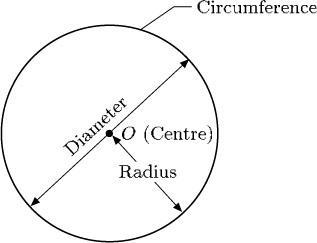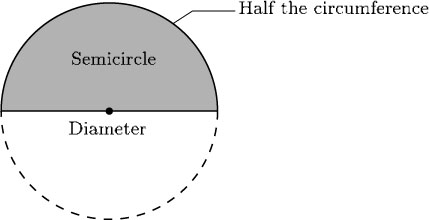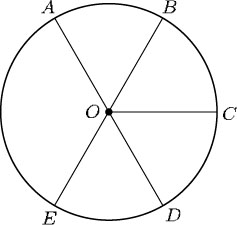Science, Maths & Technology

### Become an OU studentGeometry

Start this free course now. Just create an account and sign in. Enrol and complete the course for a free statement of participation or digital badge if available.

# 2.3 Geometric shapes – circles

All circles are the same shape – they can only have different sizes.

In a circle, all the points are the same distance from a point called the centre. The centre is often labelled with the letter O.The outside edge of a circle is called the circumference. A straight line from the centre to a point on the circumference is called a radius of the circle (the plural of radius is radii).

A line with both ends on the circumference and passing through the centre is called a diameter. Any diameter cuts the circle into two halves called semicircles.In the circle below, the lines labelled OA, OB, OC, OD and OE are all radii, and AD and BE are diameters. The points A, B, C, D and E all lie on the circumference.Although the terms ‘radius’, ‘diameter’ and ‘circumference’ each denote a certain line, these words are also employed to mean the lengths of those lines. So it is common to say, for example, ‘Mark a point on the circumference’ and ‘The circumference of this circle is 7.3 cm’. It is obvious from the context whether the line itself or the length is being referred to.